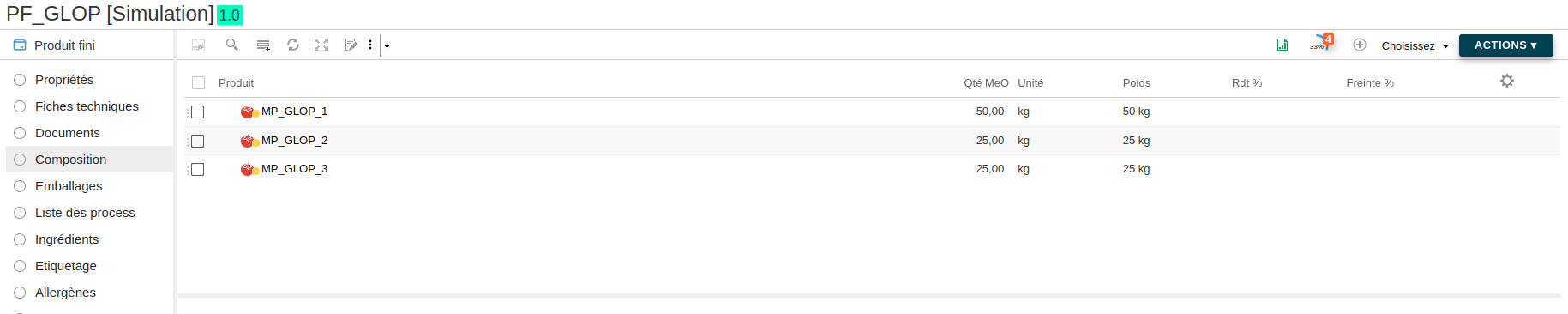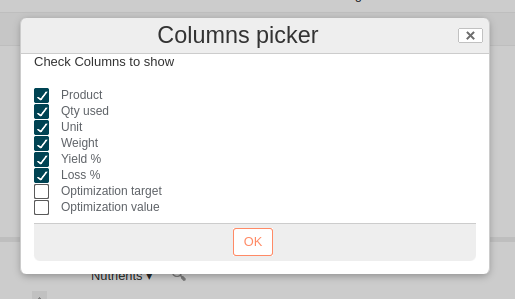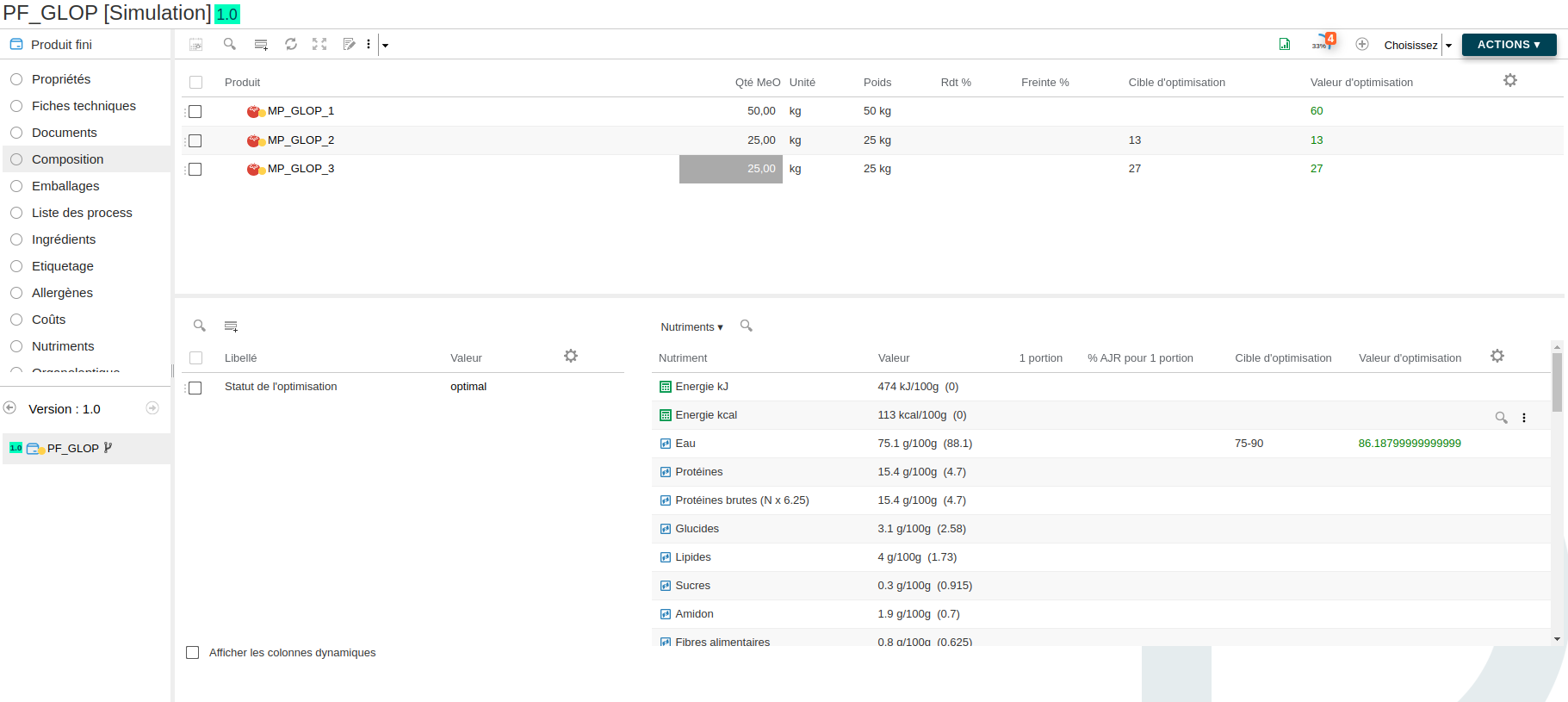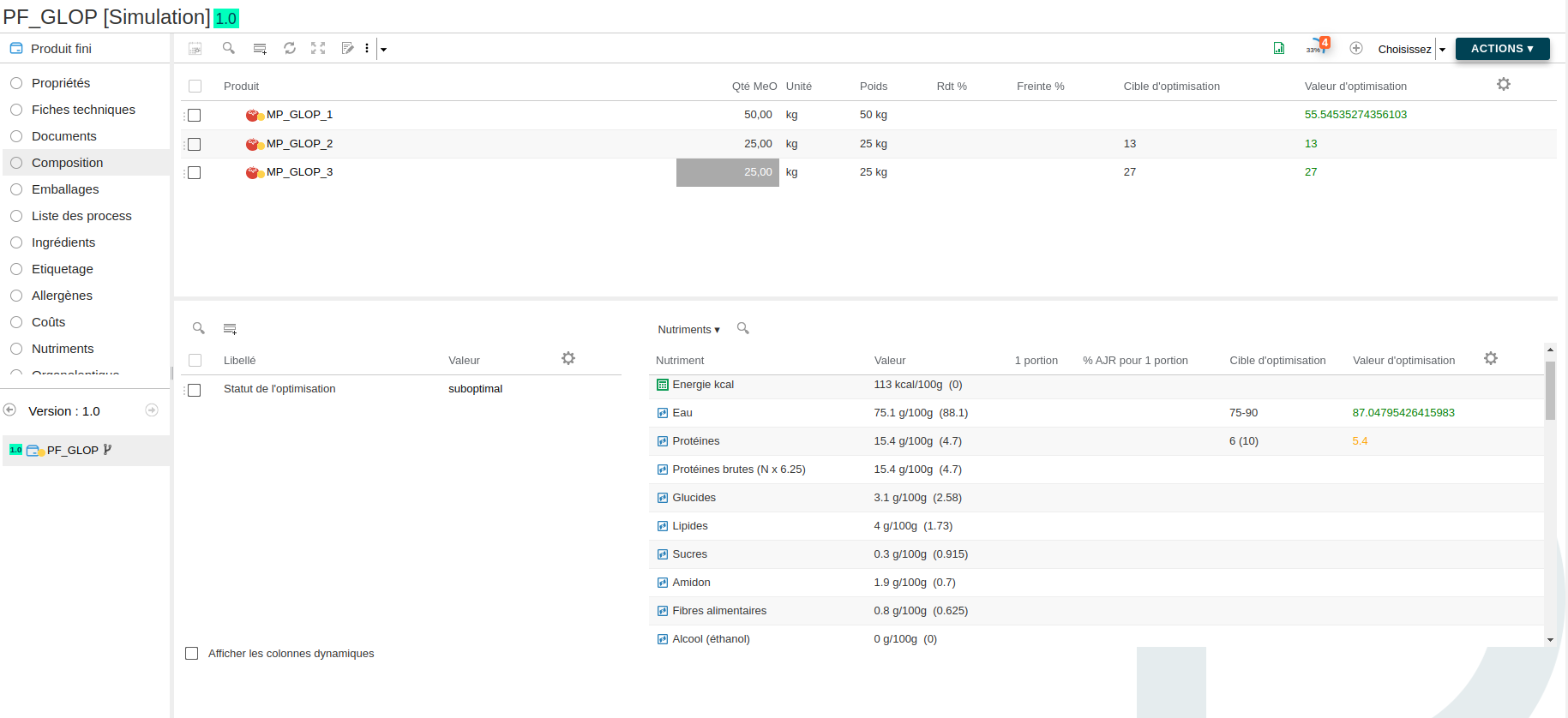# Optimisation with the Glop service

Let's start from a finished product with 3 raw materials.We desire to optimize the recipe to reach some nutrient and composition targets.

First, we need to add the columns "Optimization Target" and "Optimization value" upon composition and nutrient lists.Then we need to fill the targets in the column "Optimization Target". A target can be :

• a single value, ex: 58.2
• 2 values separated with a ";", ex: 44;48, which are the min and max (negative values are accepted)
• completed by a tolerance between parentheses, ex: 58.2 (10) ou 44-48 (15), which is the tolerance in %
• empty, which means there is no constraint for that row

Then we need to create the SPEL formula.

This is the formula used for this case :

``````var glopData = @glop.optimize({target: {var:  cost['workspace://SpacesStore/db597446-3458-405a-96d1-09b9593df1bb'], task: "min"}, constraints: {
{list: compoList},
{list: nutList}}});

setGlopData(#glopData);
glopData.getStatus();
``````

Here is the result :We can see that the system found a solution in the columns "Optimization target".

By default, the system will try to find a solution using the same amount of total quantity in the recipe. That is why the total quantity of the solution is still 100 kg.

It is possible to add a constraint on the recipe total quantity by adding the formula :

``````{var:"recipeQtyUsed", min:90,max:90}
``````

## Tolerance application

When the system cannot find a solution, it is possible to apply tolerances (in %) on the constraints.

• From the SPEL formula : `{list: compoList, tol:10}` (here, the tolerance is applied for the whole list)
• From the column "Optimization target" : `6 (10)` (here, the tolerance is only applied for the row)

Here is the result :We can see the "Proteins" optimization value is orange because the system had to applied the 10% tolerance to find a solution.

The optimization status is then "suboptimal".